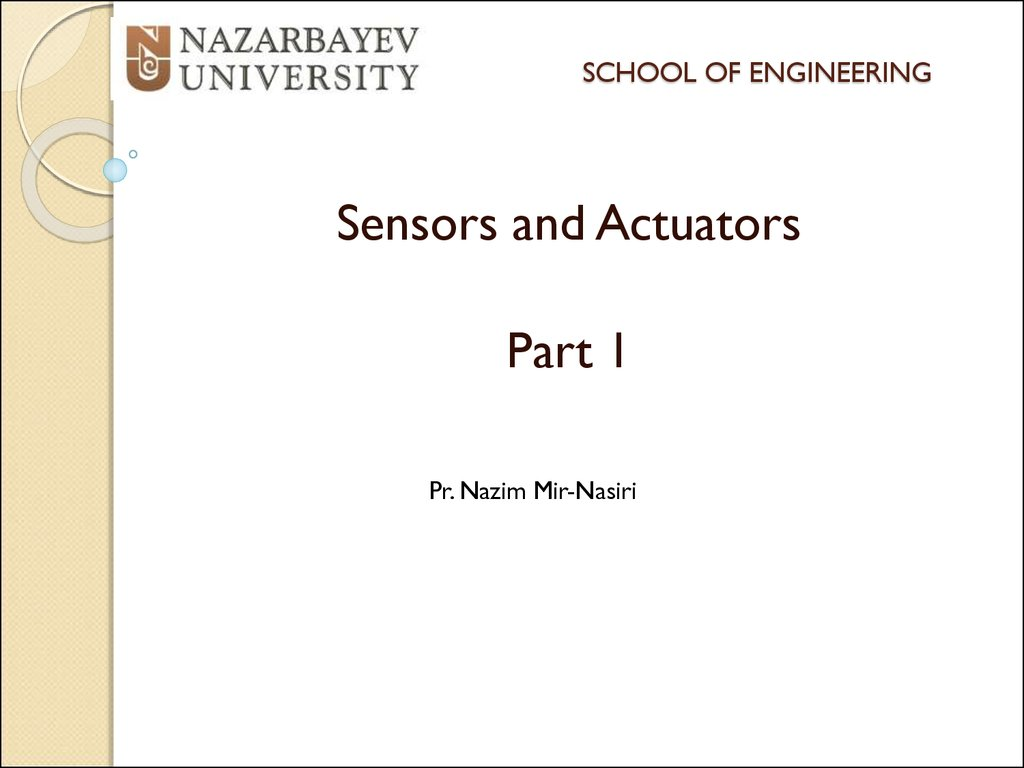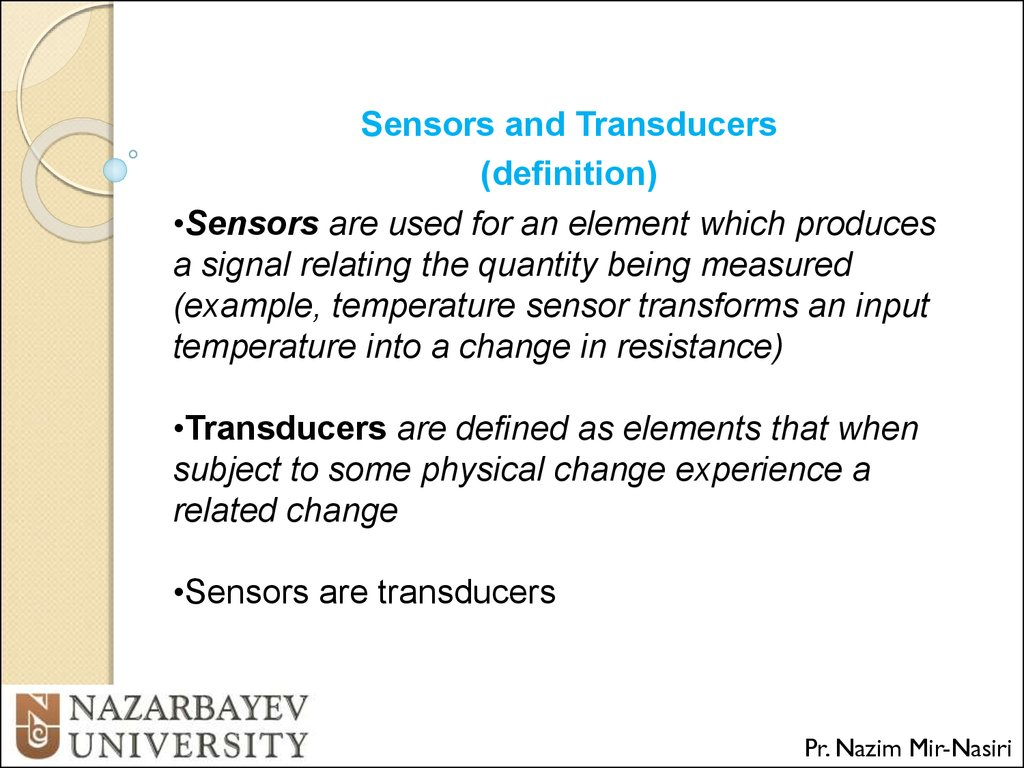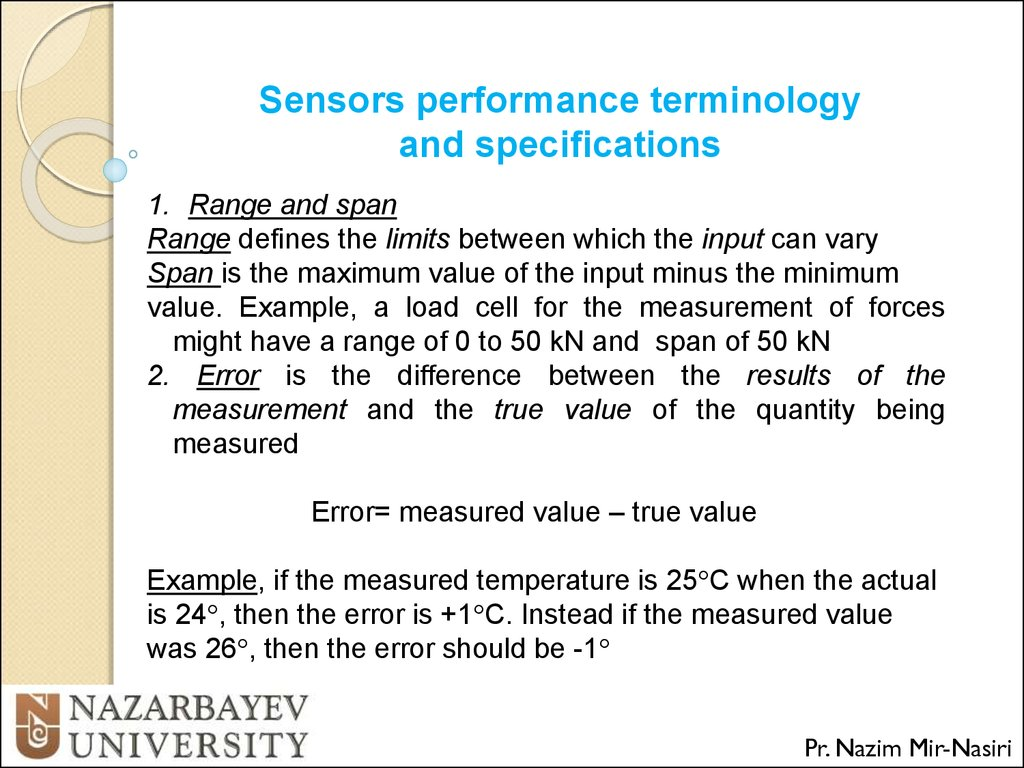# Sensors and actuators

## 1. SCHOOL OF ENGINEERING

Sensors and Actuators
Part 1
Pr. Nazim Mir-Nasiri

## 2.

Sensors and Transducers
(definition)
•Sensors are used for an element which produces
a signal relating the quantity being measured
(example, temperature sensor transforms an input
temperature into a change in resistance)
•Transducers are defined as elements that when
subject to some physical change experience a
related change
•Sensors are transducers
Pr. Nazim Mir-Nasiri

## 3.

Sensors performance terminology
and specifications
1. Range and span
Range defines the limits between which the input can vary
Span is the maximum value of the input minus the minimum
value. Example, a load cell for the measurement of forces
might have a range of 0 to 50 kN and span of 50 kN
2. Error is the difference between the results of the
measurement and the true value of the quantity being
measured
Error= measured value – true value
Example, if the measured temperature is 25 C when the actual
is 24 , then the error is +1 C. Instead if the measured value
was 26 , then the error should be -1
Pr. Nazim Mir-Nasiri

## 4.

Sensors performance terminology
and specifications
3. Accuracy is the extend to which the value indicated by a
measurement system might be wrong. In other words, it is the
summation of all the possible errors that are likely to occur
Example, the temperature – measuring instrument may be
specified as having an accuracy of 2 C of the true value . It
means that the reading given by the instrument can be
expected to lie within + or – 2 C of the true value
Accuracy is often expressed as a percentage of the full range
output or full-scale deflection
Example, the reading may be specified as having an accuracy
of 5% of full range output. Then if the range of the sensor is 0
to 200 the reading given can be expected to be within + or –
10 C of the true reading
Pr. Nazim Mir-Nasiri

## 5.

Sensors performance terminology
and specifications
4.Sensitivity is relationship indicating how much output you get
per unit of input, i.e. input/output relationships.
Example, a resistance thermometer may have a sensitivity
of 0.5 / C. The higher sensitivity is the better quality of it.
.
This term is also frequently used to indicate the sensitivity
to inputs other than being measured, i.e. environmental
changes such environmental temperature or fluctuation the in
the mains voltage supply. Example, the pressure transducer
may have a temperature sensitivity of 0.1 % of the reading per
C change in temperature.
Pr. Nazim Mir-Nasiri

## 6.

Sensors performance terminology
and specifications
5. Hysteresis error is due to the fact that transducers can give
different outputs from the same value of quantity being
measured according to whether that value has been reached
by a continuously increasing or decreasing change . It is the
maximum difference in output for increasing and decreasing
values
Pr. Nazim Mir-Nasiri

## 7.

Sensors performance terminology
and specifications
6. Non-linearity error is due to the fact that most of the
transducers have nonlinear relationships between the output
and input. However, a linear relationship is assumed in many
cases. The error is defined as the maximum difference from the
linear behavior.
Various methods are used for the numerical expression of the
non-linearity error . The error is generally quoted as a
percentage of the full range output.
Example, 0.5 % of the full range.
Pr. Nazim Mir-Nasiri

## 8.

Sensors performance terminology
and specifications
6. Non-linearity error
a. The error is defined as the
difference between the curve
and the straight line joining the
output values at the end points
of the range
Pr. Nazim Mir-Nasiri

## 9.

Sensors performance terminology
and specifications
6. Non-linearity error
b. The error is defined as the
difference between the curve
and the straight line drawn by
using the method of least
squares that defines the best fit
line when all data values are
considered equally likely to be
in error.
Pr. Nazim Mir-Nasiri

## 10.

Sensors performance terminology
and specifications
6. Non-linearity error
c. The error is defined as the
difference between the curve
and the straight line drawn by
using the method of least
squares that defines the best fit
line which passes through the
zero point.
Pr. Nazim Mir-Nasiri

## 11.

Sensors performance terminology
and specifications
7. Repeatability/reproducibility of a transducer are used to
describe its ability to give the same output for repeated
application of the same input value. It is usually expressed as a
percentage of the full output.
Repeatability =
Pr. Nazim Mir-Nasiri

## 12.

Sensors performance terminology
and specifications
8. Stability of a transducer is its ability to give the same output
when used to measure a constant input over a period of time.
The term drift is often used to describe the change in output
that occurs over time. Can be expressed as a percentage of
the full range output.
The term zero drift is used for the change that occur in the
output when there is zero input.
Pr. Nazim Mir-Nasiri

## 13.

Sensors performance terminology
and specifications
9. Dead band/time of a transducer is the range of input values
for which there is no output.
Example, bearing friction in a flow meter using a rotor might
mean that there is no output until the input has reached a
particular velocity threshold .
The dead time is the length of time from the application of an
input until the output begins to respond and change.
Pr. Nazim Mir-Nasiri

## 14.

Sensors performance terminology
and specifications
10. Resolution.
When the input varies continuously over the range, the output
signals for some sensors may change in small steps.
The resolution is the smallest change in the input value that will
produce an observable change in the output
Example, in wire-wound potentiometer the output going up in
steps as the potentiometer slides mover from one wire turn to
the next. The resolution of it can be specified, say, 0.5 or
percentage of the full-scale deflection.
For a sensor giving a digital output the smallest change in
output is 1 bit. The higher number of bits the better is the
resolution, i.e. it is smaller. The sensor with data word of N bits
digital or a total of 2N decimal, the resolution is 1/ 2N
Pr. Nazim Mir-Nasiri

## 15.

Sensors performance terminology
and specifications
11. Output impedance
When a sensor giving an electrical output is interfaced with an
electronic circuits it is necessary to know the output impedance
since this impedance is being connected in either series or
parallel with that circuit.
The inclusion of the sensor can significantly modify the
behavior of the system to which it is connected.
Pr. Nazim Mir-Nasiri

## 16.

Sensors performance terminology
and specifications
Example of the specification of a stain gauge pressure sensor
Pr. Nazim Mir-Nasiri

## 17.

Static and Dynamic Characteristics of
Sensors
•The static characteristics are the values given when steadystate conditions occur, i.e. the values given when the
transducer has settled after having received some input
• The dynamic characteristics refer to the behavior (or system
response) between the time that the input value changes and
the time when the output settles down to the steady-state value
Example, the input might be a step input when the input is
suddenly changed from 0 to a constant value, or a ramp input
when the input is changed at a steady rate, or a sinusoidal
input of a specified frequency
Pr. Nazim Mir-Nasiri

## 18.

Static and Dynamic Characteristics of
Sensors
•Response time is the time which
elapses after a constant step
input is applied to the transducer
up to the point at which the
transducer gives an output
corresponding to some
percentage, e.g. 95% of the input
.
Example, mercury-in-glass
thermometer is put into a hot
liquid there can be as much as
100 s elapsed before the
thermometer indicates 95% of the
actual temperature of the liquid.
Pr. Nazim Mir-Nasiri

## 19.

Static and Dynamic Characteristics of
Sensors
•Time constant is the 63.2%
response time. It is a measure of
the inertia of sensor and so how
fast it will react to changes in its
input. The bugger the time
constant the slower will be its
reaction to a changing input
• Rise time is the time taken for
the output to rise to some
specified percentage of the
from 10% to 90% of the steadystate
Pr. Nazim Mir-Nasiri

## 20.

Static and Dynamic Characteristics of
Sensors
•Settling time is the time taken for the output to settle to within
some percentage, e.g. 2% of the steady-state value
Example. Consider the following data which indicates how an
instrument reading changed with time, being obtained from a
thermometer plunged into a liquid at time t=0 s. The 95%
response time is required
Pr. Nazim Mir-Nasiri

## 21.

Static and Dynamic Characteristics of
Sensors
Example. Fig. shows the graph of
how the temperature indicated by the
thermometer varies with time. The
steady-state value is 55 C and so,
since 95% of 55 is 52.25, the 95%
repose time is about 228 s.
Pr. Nazim Mir-Nasiri

THANK YOU